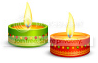Grab discount upto 30% & valuable freebies under Saral Gyan Dussehra - Diwali Offer 2020. Click here for details.SARAL GYAN DUSSEHRA - DIWALI OFFER OF THE YEARMULTIBAGGER SMALL / MID CAPS & LONG TERM INVESTING REPORT >>>  FREE DOWNLOAD

PAST PERFORMANCE >>> HIDDEN GEMS, VALUE PICKS & WEALTH-BUILDER >>>  VIEW / DOWNLOAD

SUBSCRIPTION:        FREE SUBSCRIPTION      |      PAID SUBSCRIPTION     |      PAYMENT OPTIONS

# Determining Rate of Return

Determining Rate of Return

Probably the most basic calculation for investors is return on investment. Total return includes capital appreciation and income components, and assumes all income distributions are reinvested. If you automatically reinvest distributions such as interest or dividends, total return is calculated by taking the difference in an investment portfolio's ending and beginning balance, and dividing that difference by the beginning balance. In formula, it would look like this:

Total Return:

(Ending Balance [EB] - Beginning Balance [BB])

For example, Pankaj started with an investment of Rs.10,000. After five years, his portfolio's value increased to Rs.12,000. He can determine his portfolio's total return as follows: (Rs.12,000 – Rs.10,000) / Rs.10,000 = 0.20, or 20%. Therefore, Pankaj can say his Rs.10,000 has increased by 20%.

To annualize this total return, you'll need to calculate the compound annual return.

For example, Satish also originally invested Rs.10,000. However, it took his portfolio only two years to grow to Rs. 12,000. If you measure the performances of both Pankaj's and Satish's portfolios by using the formula above, both increased by 20%. To take the difference in time into consideration, calculate the compound annualized rate of return (you will need a calculator that can raise to powers to calculate this).

Compound Annualized Rate of Return = [(EB / BB) ^ (1 / # of years) - 1]

Using this formula to calculate Pankaj's annual compound return, we take Rs. 12,000 / Rs.10,000 = 1.2. Then, we raise 1.2 to the 1/5 (or 0.20) power, giving us 1.03714. Subtract out 1, and we have 0.03714, or 3.714%, which is Pankaj's annualized return. Satish's portfolio, on the other hand, performed much better, earning 9.54% on average every year. Of course, two different investments should not be judged solely on performance results for short periods of time or for different time periods. The risk of the portfolio must also be considered.